Physics
Easy

Question

# The displacement time graph of a particle executing S.H.M. is as shown in the figure: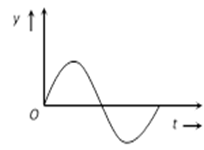The corresponding force-time graph will be:

##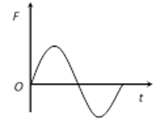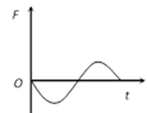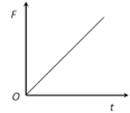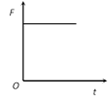Hint:

## The correct answer is:### According to the Simple harmonic equation of motion, the force is directly proportional to the negative of displacement.The simple harmonic equation of motion is defined as,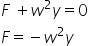As  is always positive, Therefore the force is directly proportional to the negative displacement. Hence, the force-time graph will be invert of the displacement-time graph.Hence, option (B) is the correct answer.#### With Turito Foundation.#### Get an Expert Advice From Turito.